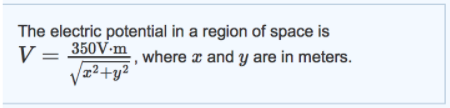# Problem: The electric potential in a region of space is V=350V?mx2+y2?, where x and y are in meters.Part AWhat is the strength of the electric field at (x,y) = (2.3m, 2.9m)?Express your answer using two significant figures.Part BWhat is the direction of the electric field at (x,y) = (2.3m, 2.9m)? Give the direction as an angle ccw from the positive x-axis.Express your answer using two significant figures.

###### FREE Expert Solution

The electric field is given by:

$\overline{){\mathbf{E}}{\mathbf{=}}{\mathbf{-}}{\mathbf{\nabla }}{\mathbf{V}}{\mathbf{=}}{\mathbf{-}}{\mathbf{\left(}}\frac{\mathbf{\partial }}{\mathbf{\partial }\mathbf{x}}\stackrel{\mathbf{^}}{\mathbf{i}}{\mathbf{+}}\frac{\mathbf{\partial }}{\mathbf{\partial }\mathbf{y}}\stackrel{\mathbf{^}}{\mathbf{j}}{\mathbf{\right)}}{\mathbf{V}}}$

Vector Magnitude:

$\overline{)\mathbf{|}\stackrel{\mathbf{⇀}}{\mathbit{A}}\mathbf{|}{\mathbf{=}}\sqrt{{{\mathbit{A}}_{\mathbit{x}}}^{\mathbf{2}}\mathbf{+}{{\mathbit{A}}_{\mathbit{y}}}^{\mathbf{2}}}}$

Direction:

$\overline{){\mathbf{tan}}{\mathbit{\theta }}{\mathbf{=}}\frac{{\mathbit{A}}_{\mathbit{y}}}{{\mathbit{A}}_{\mathbit{x}}}}$

96% (250 ratings)###### Problem Details

The electric potential in a region of space is V=350V?mx2+y2?, where x and y are in meters.Part A

What is the strength of the electric field at (x,y) = (2.3m, 2.9m)?